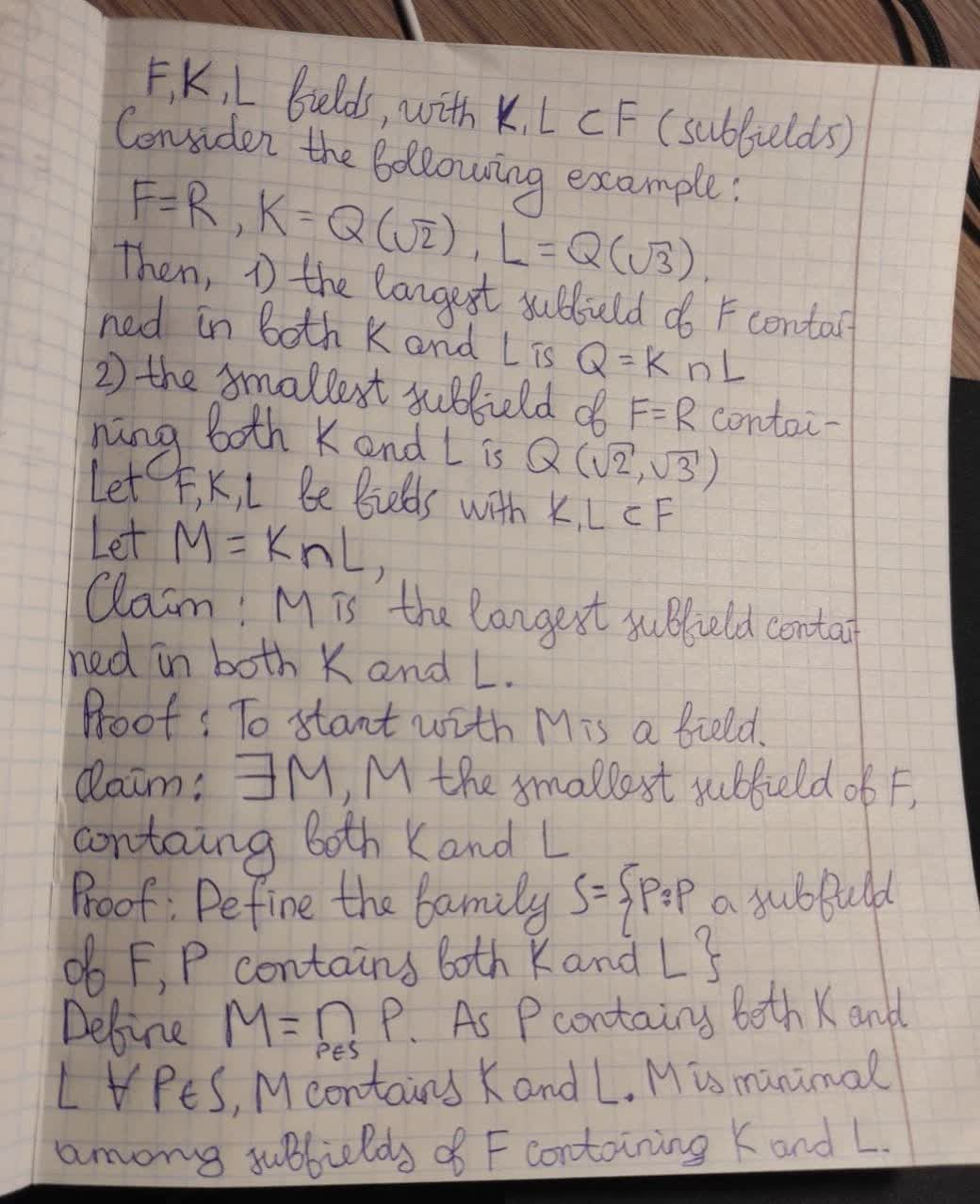# Let F be i field with subfields K,L. Prove that there is a largest subfield of F contained in both K and L, and a smallest subfield of containung both K and L.Rui Baldwin 2020-12-15 Answered
Let F be i field with subfields K,L. Prove that there is a largest subfield of F contained in both K and L, and a smallest subfield of containung both K and L.
You can still ask an expert for help

• Questions are typically answered in as fast as 30 minutes

Solve your problem for the price of one coffee

• Math expert for every subject
• Pay only if we can solve itMitchel Aguirre

To get the general picture, first consider a simple example.
F,K,L fields, with K,L sub F (subfields)
Consider the followinf wxample: $F=\mathbb{R},K=\mathbb{Q}\left(\sqrt{2}\right),L=\mathbb{Q}\left(\sqrt{3}\right).$ Then,
1) the largest subfield of F contained in both $K\phantom{\rule{1em}{0ex}}\text{and}\phantom{\rule{1em}{0ex}}Lis\mathbb{Q}=K\cap L$
2) the smallest subfield of $F=\mathbb{R}$ containing both $K\phantom{\rule{1em}{0ex}}\text{and}\phantom{\rule{1em}{0ex}}Lis\mathbb{Q}\left(\sqrt{2},\sqrt{3}\right)$
Now consider the general case. The largest (sub)field of F contained in both K and L is clearly $K\cap L$. See details in the proof.
Let F,K,L be fields, with $K,L\subset F$ (subfields)
Let $M=K\cap L$,
Claim: M is the largest sunfield contained in both K and L.
Proof: To start wi M is a field.
So, a subfield of $F,S\subset K,S\subset L⇒S\subset K\cap L=\left(M\right)$
This, shows that M=K cap L is the maximal subfield of F with respect to this property. Hence the claim is true.
To show the existence of the smallest subfield containing K and L , proceed as in the proof.. Note that we cant work with the union of K and L , as the union of two fields need not be a field. So, a different approach is needed.
Let F,K,L be fields with $K,L\subset F$ (subfields)
Claim: $\mathrm{\exists }m$, m the smallest subfield of F, containing both K and L (as subfields)
Proofs: Define family S={P:P a subfield of F, P contains both K and L}
To start with, note that S is non - empty family, as F in S
Completing the proof that M , as defined, is the smallest subfield of F containing both K and L.
Proof: Define the family S={P:P a subfield of F, P contains both K and L}
To start with, note that S is non - empty family, as$F\in S$
Define $M=\bigcap _{P\in S}P.$
As P contains both $K\phantom{\rule{1em}{0ex}}\text{and}\phantom{\rule{1em}{0ex}}L\mathrm{\forall }P\in S$, M contains both K and L.
M is the intersection of all such P, M is the minimal among all subfields of F containg both K and L